# Common Core: 1st Grade Math : Use Properties of Operations to Add and Subtract: CCSS.MATH.CONTENT.1.OA.B.3

## Example Questions

1 2 3 5 Next →

### Example Question #41 : Common Core Math: Grade 1

If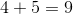, then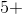__________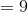Explanation:

This is an example of commutative property. Commutative property of addition means that you can change the order of the smallest two numbers when adding, and it will always equal the biggest number.

### Example Question #1331 : How To Add

Which math problem below equals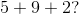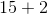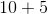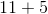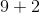Explanation:

We can add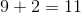. So the math problem becomes. Bothand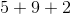equal### Example Question #41 : Use Properties Of Operations To Add And Subtract: Ccss.Math.Content.1.Oa.B.3

Which math problem below equals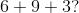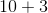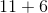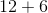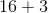Explanation:

We can add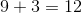. So the math problem becomes. Bothand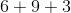equal.

### Example Question #44 : Common Core Math: Grade 1

Which math problem below equals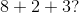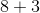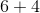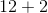Explanation:

We can add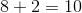. So the math problem becomes. Both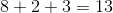and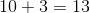### Example Question #45 : Common Core Math: Grade 1

Which math problem below equals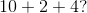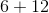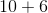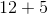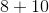Explanation:

We can add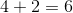. So the math problem becomes. Both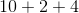andequal### Example Question #41 : Understanding And Applying Properties Of Operations And The Relationship Between Addition And Subtraction

Which math problem below equals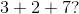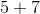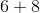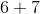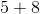Explanation:

We can add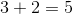. So the math problem becomes. Both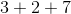andequal### Example Question #41 : Common Core Math: Grade 1

Which is an example of commutative property?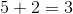and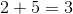and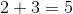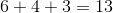andand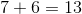andExplanation:

The commutative property says that you can add the numbers in any order and still get the same answer.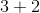and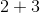both equal### Example Question #41 : Common Core Math: Grade 1

Which is an example of associative property?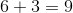and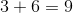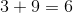and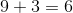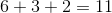and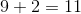and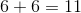andExplanation:

The associative property says that you can group a set of numbers in a series, and still get the same answer. If we group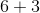to get, and then addwe get a sum of1 2 3 5 Next →

### All Common Core: 1st Grade Math Resources